# Definite double integral of sin(y²)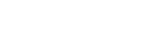I’ll be honest: I had no idea how to do this at first, but then I thought that it was probably easier than I could imagine. What most likely blocks you when you see this is the function inside the integral, which, as we saw here, is a special function, meaning its antiderivative is not an elementary function. This is the only way to express the result: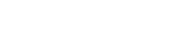The function S is not a function we know, it could have also been A, K, G.

But the fact that the result is just S(x) could actually be good for the resolution of our double integral. Let’s see what we can do:

First of all, we calculate the inner integral, which is with respect to y. Let’s first treat it as an indefinite integral: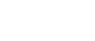This is exactly what we have just seen, the only difference being the ‘y‘;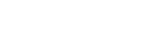Now we can plug in the bounds of integration, x and 1: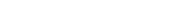At this point you may wonder what S(1) is. This is what I asked myself too, but then I thought that maybe there was no need to evaluate it, so I just kept going.

This is the result of the inner integral, and now we have to integrate it with respect to x (this is the outer integral with bounds 0 and 1):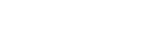Since it is a difference, we can apply linearity and obtain two integrals: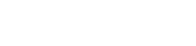Inside the first integral we have a constant, because there is not the variable x, therefore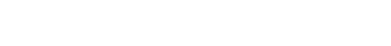and so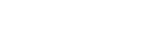Now, what on earth is the integral of S(x)? Again, despite how it looks, it’s actually very simple using integration by parts, the exact same way we found the integral of ln(x).

It would make no sense to let dv be S(x), since that way we would have to integrate it, but that’s exactly what we are trying to do; instead, we can let u=S(x) so that we can take the derivative: if the integral of sin(x²) is S(x), the derivative of this last function is the function inside the integral. Therefore,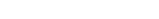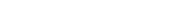As you probably know, the result is uv-int(vdu);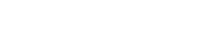Remember the bounds, since it’s a definite integral. We can plug them in the first part: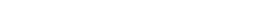and so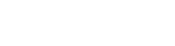But wait: if you remember, we hadand what we’ve just found is this integral, so we can replace it with the result: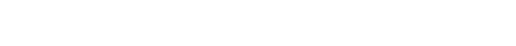As you can see, S(1)-S(1)=0. How amazing is this?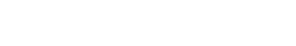At this point we are left with this integral, which is actually very easy to evaluate performing a u-substitution:

let’s let u=x², so that its derivative will cancel out with the x in front of sin: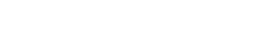Don’t forget that the integral is with respect to x, which means the bounds are values of x. Since we are performing the u-substitution we need to find the bounds of u; since u=x², u1 (lower bound)=x1²=0, and u2 (upper bound)=x2²=1.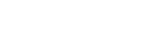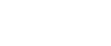The x‘s cancelled out and the 1/2 was brought to the front of the integral (linearity). This is one of the “fundamental” integrals: the antiderivative of sin(x) is -cos(x).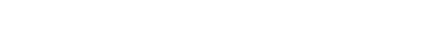If you set your calculator to rad you’ll get that cos(1) is approximately 0.54, therefore the final result is (-0.54+1)/2=0.46/2=0.23.

Overall,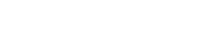or, if you prefer,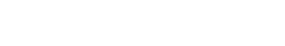That’s it! I hope you liked this post and that you found it interesting! If you did, give it a like and subscribe to stay updated!

Join 31 other subscribers.